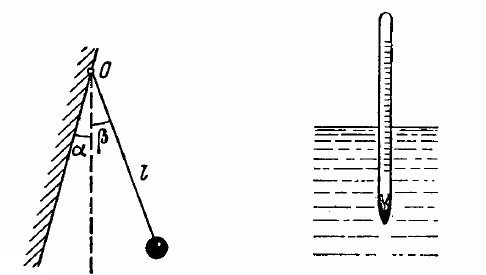# ball is suspended by a thread of length l at the point 0 on the wall, forming a small angle a with the vertical (Fig. 4.1). Then Fig. 4.1, Fig. 4.2. t...

ball is suspended by a thread of length l at the point 0 on
the wall, forming a small angle a with the vertical (Fig. 4.1). Then
Fig. 4.1, Fig. 4.2.
the thread with the ball was deviated through a small angle 13(l > a)
and set free. Assuming the collision of the ball against the wall to
be perfectly elastic, find the oscillation period of such a pendulumAnonymous User Physics Oscillation 01 May, 2020 61 views